﻿ Introduction to Polygons

#Introduction to Polygons

You may want to review:

DEFINITION polygon
A polygon is a closed figure in a plane made by joining line segments, where each line segment intersects exactly two others.

Note: Strictly speaking, a polygon does not include its interior (the space inside the polygon).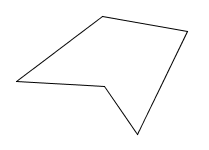A polygonNot closed;
not a polygon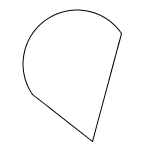Not made of line segments;
not a polygonLine segment intersects more than two others;
not a polygon

Polygons are usually classified according to how many sides they have:

DEFINITIONS names of polygons with various numbers of sidesA triangle is a polygon with $\,3\,$ sides.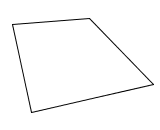A quadrilateral is a polygon with $\,4\,$ sides.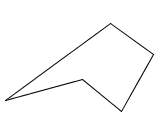A pentagon is a polygon with $\,5\,$ sides.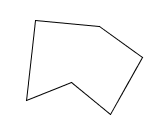A hexagon is a polygon with $\,6\,$ sides.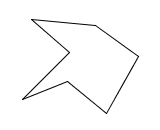A heptagon is a polygon with $\,7\,$ sides.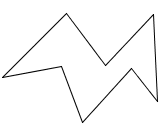An octagon is a polygon with $\,8\,$ sides.A nonagon is a polygon with $\,9\,$ sides.A decagon is a polygon with $\,10\,$ sides.

More generally, a polygon with $\,n\,$ sides can be called an $\,n\,$-gon.

For example, a polygon with $\,27\,$ sides can be called a $\,27$-gon.

DEFINITION vertex of a polygon; vertices

The vertices of a polygon are the points where its sides intersect. The singular form of vertices is vertex.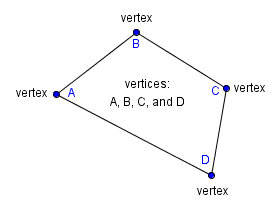## Naming PolygonsWhen naming polygons, the vertices must be listed in consecutive order. For example, the polygon above could be named:

• polygon $\,ABCD\,$ (start with $\,A\,,$ move clockwise)
• polygon $\,ADCB\,$ (start with $\,A\,,$ move counter-clockwise)
• polygon $\,BCDA\,$ (start with $\,B\,,$ move clockwise)
• polygon $\,BADC\,$ (start with $\,B\,,$ move counter-clockwise)
• polygon $\,CDAB\,$ (start with $\,C\,,$ move clockwise)
• polygon $\,CBAD\,$ (start with $\,C\,,$ move counter-clockwise)
• polygon $\,DABC\,$ (start with $\,D\,,$ move clockwise)
• polygon $\,DCBA\,$ (start with $\,D\,,$ move counter-clockwise)

More generally, when naming an $\,n$-gon, there are $\,n\,$ choices for listing the first vertex. Then, there are $\,2\,$ choices for the next vertex (moving clockwise or counterclockwise). The remaining vertices are then completely determined. Thus, there are $\,2n\,$ choices for the polygon name.

DEFINITION regular polygon
A regular polygon is a polygon whose sides all have the same length, and whose angles are all the same.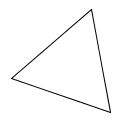a regular triangle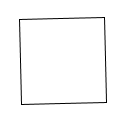a regular quadrilateral
(a square)a regular pentagona regular hexagon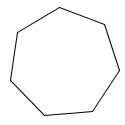a regular heptagona regular octagona regular nonagona regular decagon
DEFINITIONS rectangle, square

A rectangle is a quadrilateral whose angles are all right angles.

A square is a rectangle with all sides of equal length.

Note: Every square is a rectangle. However, not every rectangle is a square. That is, there exist rectangles that are not squares.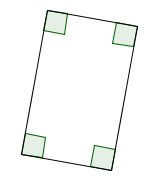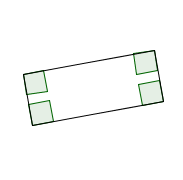Each of these is a rectangle, but not a square.

For fun, jump up to WolframAlpha and type in (say) ‘triangle’ or ‘quadrilateral’. You'll get loads of information!